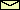# The Vertically Driven Pendulum

acceleration of gravity = 9.81 m/sec2

For instructions click on here.
For the equation of motion click on here

Suggestions for EXPERIMENTS
1. Choose length = 1 m, damping = 0.1 sec-1, and amplitude = 0.07 m. Start with an initial condition of roughly 10° and observe the behavior for different values of the frequency between 0.2 Hz to 1.2 Hz. In most cases, the initial oscillation dies out except for values around 1 Hz where the down-hanging equilibrium is no longer stable. Note, that the frequency of the excited oscillation is half the frequency of driving.
 Related topics in the lecture room: Parametric resonance.
2. Choose length = 1 m, damping = 0.1 sec-1, amplitude = 0.07 m, and frequency = 0.8 Hz. Depending on the initial condition, you will get either a strong oscillation or the oscillations decay to zero.
 Related topics in the lecture room: Parametrically excited oscillations.
3. Choose length = 1 m, amplitude = 0.3 m, frequency = 3 Hz, and arbitray nonzero damping. Start with an intial condition near 180°. You will find that the upside-down pendulum is no longer unstable. Why?
 Related topics in the lecture room: The upside-down pendulum.

© 1998 Franz-Josef Elmer,Franz-Josef doht Elmer aht unibas doht ch, last modified Saturday, June 27, 2011.